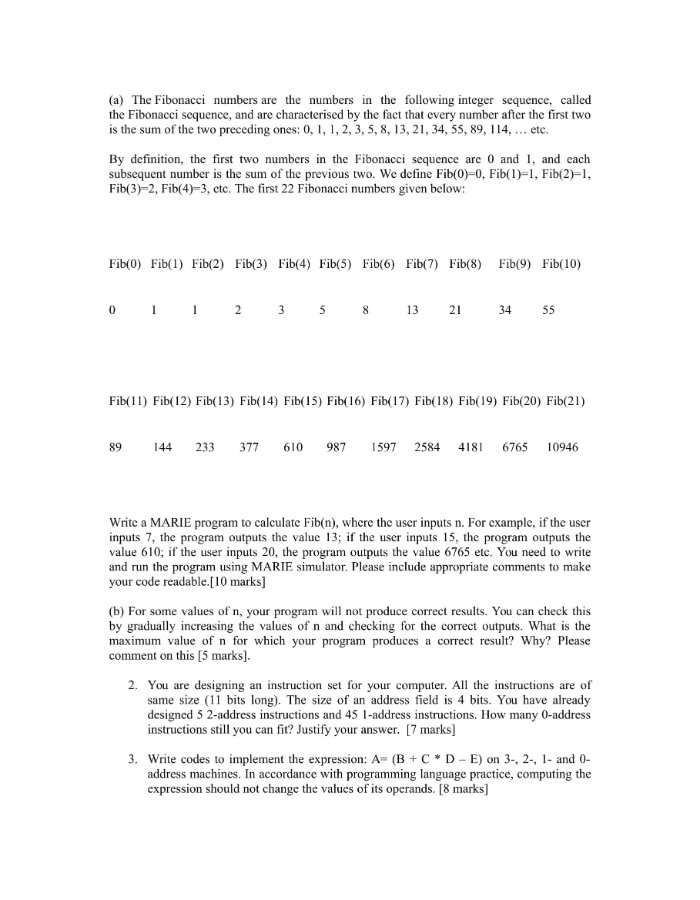# Write a MARIE Program to Calculate Fib

Home Recent Questions Write a MARIE Program to Calculate Fib

(a) The Fibonacci numbers are the numbers in the following integer sequence, called the Fibonacci sequence, and are characterised by the fact that every number after the first two is the sum of the two preceding ones: 0, 1, 1, 2, 3, 5, 8, 13, 21, 34, 55, 89, 114, … etc.